### Home > MC2 > Chapter 8 > Lesson 8.2.3 > Problem8-59

8-59.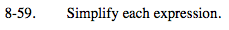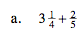Notice that the whole number and the fractions can be added separately.

$3 + \left( \frac{1}{4} + \frac{2}{5} \right)$

$3 \frac{13}{20}$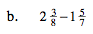You may want to convert the mixed numbers into fractions greater than one before simplifying.

$\frac{19}{8} - \frac{12}{7}$

$\frac{37}{56}$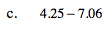Remember to line up the decimal points when adding or subtracting numbers with decimals.

−2.81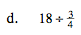When you divide a fraction, remember you are multiplying its reciprocal.

$\frac{18}{1} \left( \frac{4}{3} \right)$

24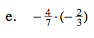Multiply.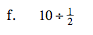See (d).

$-\frac{205}{24}$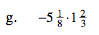Converting the mixed numbers into fractions greater than one may make this expression easier to simplify.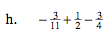Find a common denominator.